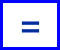# Find the Missing Number to Complete the Sum

In this worksheet, students will complete a calculation by adding in the missing numbers. It will strengthen their number skills.This content is premium and exclusive to EdPlace subscribers.Key stage:  KS 2

Curriculum topic:   Verbal Reasoning

Curriculum subtopic:   Complete the Calculation

Difficulty level:#### Worksheet Overview

Calling all number detectives!

Look at this symbol:We know it is the equals sign but what does it actually mean?

It means the same as and doesn’t just mean the answer.

Look at the following calculation:

19 = 7 + __

One of the numbers is missing.

So 19 is the same as 7 add something.

Now we have two options, we can count on from 7 to 19 or take away 7 from 19 to work out the missing number.

Following either of these options, we find that the missing number is 12.

19 = 7 + 12Let’s try another:

17 = 20 - __

In this question, the answer of 17 is the same as 20 take away something.

What do we need to take away from 20 to reach 17?

The missing number is 3.

17 = 20 - 3

Remember that equals means the same as and the answers on either side of the equals sign must be the same.

In this activity, you will be completing sums by finding the missing number.

Good luck!

### What is EdPlace?

We're your National Curriculum aligned online education content provider helping each child succeed in English, maths and science from year 1 to GCSE. With an EdPlace account you’ll be able to track and measure progress, helping each child achieve their best. We build confidence and attainment by personalising each child’s learning at a level that suits them.

Get started